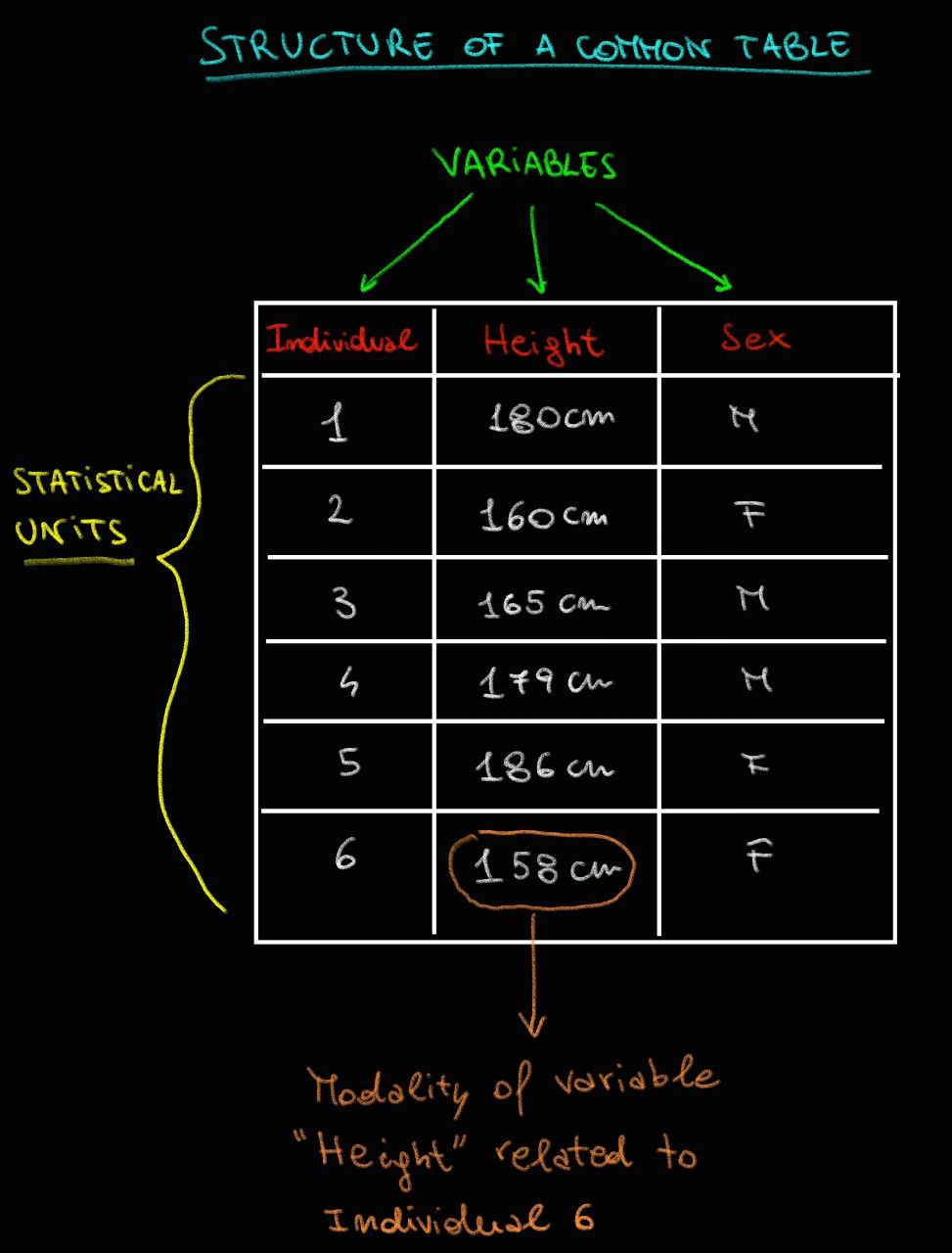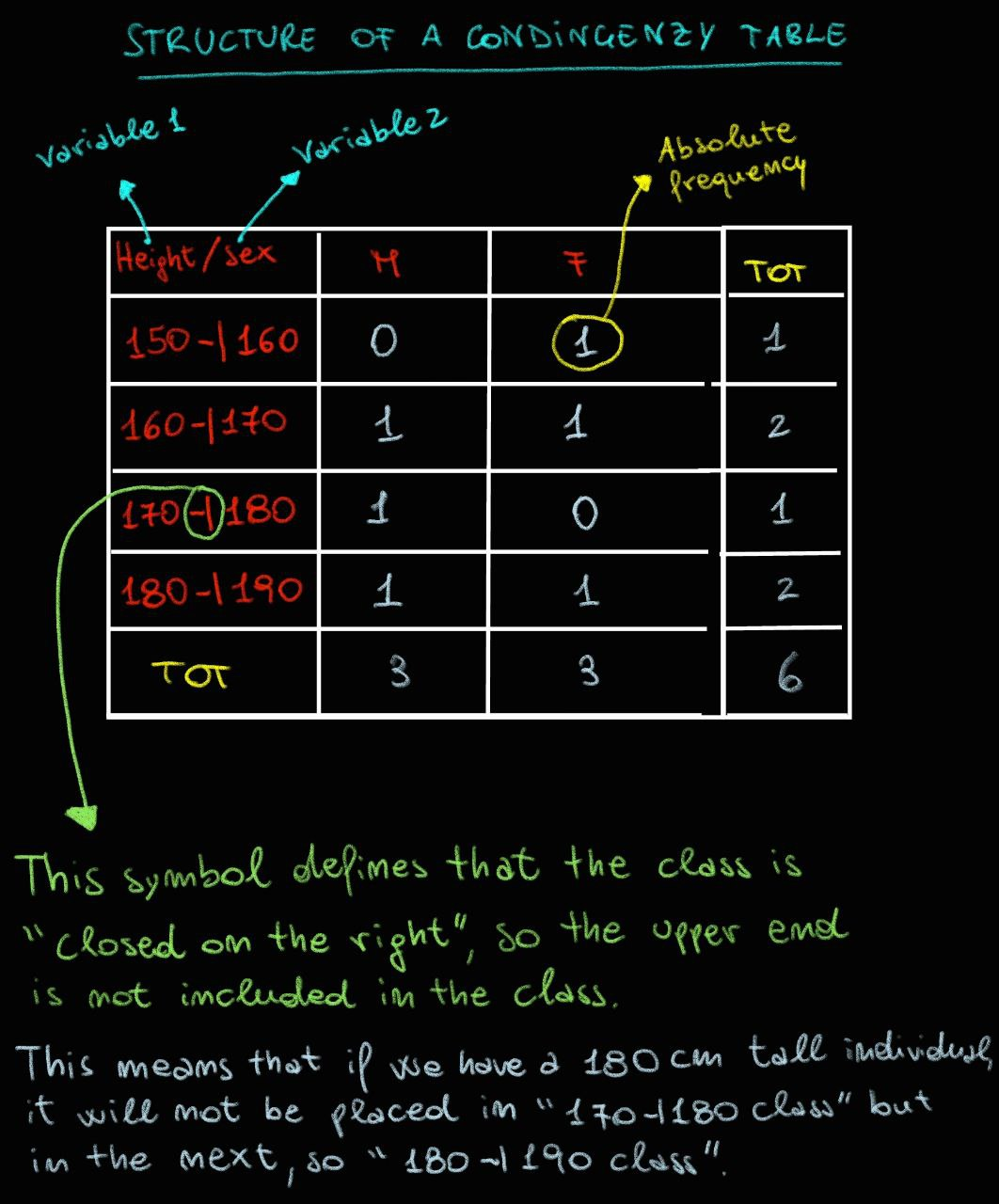Hi, how are you? Today is a beautiful day. Currently, I'm in Germany, specifically in Julich. With this post, I would like to talk to you about a subject that I'm very passionate about: statistics. I don't think it's an easy subject at all. I often forget the notions that I learn, and especially, sometimes it's not clear which statistical tool apply to a specific context. One thing is certain: Statistics is essential not only for a bioinformatician but for anyone who faces a problem that needs to be solved. Applying statistics even in daily life allows us to make more rational decisions and interpret reality, reducing the risk of falling into cognitive errors, the so-called biases.
I love definitions because they allow us to present complex concepts in a simple way. So, let's start by saying that:

Statistics is a set of methodologies that allow us to answer problems in a rational and objective way.

Let's give an example:

Suppose your friend informs you that, in their opinion, Chinese people are shorter than Italians. You are now faced with a decision: to evaluate whether your friend's statement is true or false. By taking your prejudice as a reference point, you might agree with your friend. But be careful: this decision is not rational. You have approved the idea that Chinese people are shorter than Italians based on a subjective judgment. You understand that your decision could be wrong? To objectively affirm that Chinese people are shorter than Italians and closer to the reality of the facts, it is necessary to apply statistical methods of investigation that offer us an objective answer to the problem.

Here's what I would do:

#### 1) First of all, I would take paper and pen and create an experimental design. In other words, I would try to structure the research method by answering three questions:

• What is the question I want to answer?
"Are Chinese people shorter than Italians?"

At this first level of the experimental design, we can add some key statistical terms.

With the terms "Chinese" and "Italians", I am referring to two populations of individuals. Each single individual in the population is a statistical unit. Often, studying an entire population of statistical units is difficult, if not impossible, so we try to work on a subgroup of the population, called a sample. Therefore, Chinese people constitute our population of inquiry. Each single Chinese citizen is a statistical unit, and a subgroup of the Chinese population is called a sample. Obviously, the same applies to Italians.• What kind of data do I need to answer the question asked?
To answer this question, we need to clarify some useful concepts. Data is any information, numerical or not, related to the problem at hand. Data can take different forms, such as simple strings, vectors, tables, data frames, or even multimedia.The data of interest to us are those that provide information on the values of the analyzed variables. A variable is a characteristic of a statistical unit, and its value is called a 'modality'.

Returning to our example, an individual from the sample of Italians is a statistical unit. A feature of interest for solving our problem is height. Therefore, the variable in question is 'height' and the observed value of 180 cm represents its mode.

I know, all these terms may seem boring, but they are very useful because avoid confusion during the study.At this point, it is necessary to specify that there are different types of variables.

• Qualitative or categorical variables are expressed in characters and describe the qualities of a statistical unit. They are further divided into ordinal and nominal variables. Ordinal categorical variables, as the term suggests, have an intrinsic order, although they are not numbers (for example, an individual's level of education). Nominal categorical variables, on the other hand, cannot be ordered and can only be compared in terms of equality. For example, hair color. The color blonde cannot be described as greater or lesser than the color black; we can only say that the color blonde is different from the color black.

• Quantitative or numerical variables are expressed through numbers and describe a quantity of a statistical unit. They are further divided into discrete and continuous. Discrete numerical variables are used to express a count, for example, the number of books read by an individual in a year is effectively a discrete numerical variable. This type of variable has numerical values that are discrete (e.g. 1, 2, 3, etc.). Continuous numerical variables express a measurement relative to a statistical unit. For example, an individual's height is a continuous numerical variable because its mode is a measurement indicated by a numerical value that can be continuous (e.g. 180.00 cm, 180.01 cm, 180.02 cm, etc.).Therefore, in light of what has been written above, we can say that in order to solve our question it is useful to have data that includes information on the height of individuals in the samples under examination. Obviously, this data must be numerical and it would be very useful to report it in the form of a table, as shown in the image below.Tables are very useful because they allow us to contain information in a compact way. Moreover, from a computational methods perspective, it is easier to manipulate and extract a data point that is presented in a table rather than in a vector or a list. Generally, in a table, the rows are the individual statistical units and the columns are the variables, and in each cell, we find the corresponding value for the variable in the column and the statistical unit in the row.

There is also a particular type of table in statistics called a "Contingency Table". This type of table is very useful when we want to compare the values of two variables simultaneously. For example, suppose we have information about the "sex" variable and the "height" variable for each statistical unit. In this case, it can be useful to consider the combined values of the two variables. So, we place the values of the "height" variable in the rows and the values of the "sex" variable in the columns. In the cells, we insert the relative or absolute frequency values (don't worry, we will discuss frequencies more in a dedicated article). This is how we obtain a contingency table, just as shown in the image below.Before moving on to the third question, I want to reveal a very useful trick when working with large datasets. In fact, when working with many statistical units and therefore having many values to manage, it is possible to group them into classes. However, this causes us to lose some information, so we must carefully evaluate whether it is worthwhile to classify the values, just like in the first column of the contingency table above. Sometimes, however, it can be an acceptable compromise.

• What statistical method can help me solve my question?

This last point is extremely delicate and requires careful evaluation and experience. One should not rush and should not hesitate to ask those with more experience, or search online for how others have solved similar problems.

For example, to answer the question "Are Chinese people shorter than Italians?", one can use a statistical test called the t-test to compare the average heights of representative samples of Chinese and Italians. Then, inferential statistical methods need to be applied, which allow us to infer the results obtained on our samples to the entire population of individuals.

Furthermore, in this phase it is necessary to clarify which factors could affect the results and therefore which ones need to be taken into account. In our specific case, it is important to note that this comparison between averages may not be appropriate for various reasons, including the fact that height differences between groups can be influenced by biological, environmental, socio-economic, and cultural factors.

Finally, I would like to remind you that a good statistical study should include a descriptive analysis of the available data. This allows us to describe in a simple and intuitive way the amount of data we have available for analysis. This is possible thanks to the use of different summary indices, such as indices of position, variability, and shape of the distribution. But we will talk more about this in another post.

#### 3) Now all that remains is to proceed with the statistical analyses that I have decided to perform during the construction of the experimental design to answer the question.

Therefore, what has been said above serves to make you understand two important things: 1) Statistics is a very useful tool that allows us to answer questions, even very complex ones, objectively, rationally, and therefore closer to reality. 2) When we want to answer a question using the statistical method, it is necessary to follow a precise flow of action. We start from the creation of the experimental design, then move on to sampling, and finally apply the most useful statistical methods to solve the problem. Each of these steps requires patience, attention, and study, but in the end, we can truly say with greater, albeit not total, certainty about the world around us.

Thank you for reading this article to the end. I hope you enjoyed it and, most importantly, found it useful. I would also like to remind you that what I have written is the result of personal research that I have carried out independently, using various resources found in textbooks and online. If you have any additions or corrections to make, I am more than happy to consider them, as they help me improve. After all, making mistakes is human, and it's part of the learning process.

Bye and see you soon.

### Code section:

``````# Variable 1:
height <- c(180, 160, 165, 179, 186, 158)
# Variable 2:
sex <- c("M", "F", "M", "M", "F", "F")
# Create normal table:
df <- data.frame(height, sex)
# Print DataFrame:
print(df)

# Create a Condingency table
# Divide height values in class
breakpoints <- c(150, 160, 170, 180, 190)
height_classes <- cut(height, breakpoints, right = FALSE)